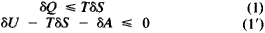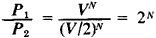# Thermodynamics, Second Law of

The following article is from The Great Soviet Encyclopedia (1979). It might be outdated or ideologically biased.

## Thermodynamics, Second Law of

a principle establishing the irreversibility of macroscopic processes taking place at a finite rate. In contrast to purely mechanical (frictionless) or electrodynamic (with no liberation of Joule heat) reversible processes, the processes with heat exchange at a finite temperature difference (that is, taking place at a finite rate) and with friction, diffusion of gases, expansion of gases into a vacuum, the liberation of Joule heat, and so forth are irreversible, that is, these processes can occur spontaneously only in one direction.

Historically speaking, the second law of thermodynamics originated from the analysis of the work done by heat engines (S. Carnot, 1824). There are several equivalent formulations of the second law of thermodynamics. The actual name “second law of thermodynamics” was given by R. Clausius, who also advanced what is historically the first formulation of the law (1850): a process in which heat flows spontaneously from colder bodies to warmer bodies is impossible. This concept of spontaneous flow should not be understood in a narrow sense: not only is such a direct flow impossible, but it cannot be brought about with the aid of machines or devices without bringing about some changes in the surroundings. In other words, it is impossible to bring about a process whose sole result would be to effect a transfer of heat from a colder body to a warmer body. If such a process were possible (in violation of Clausius’ proposition), it would then be possible to obtain two reservoirs of different temperatures by dividing one heat reservoir in two and transferring heat from one reservoir half to the other. This would in turn make it possible to create a Carnot cycle and to obtain mechanical work with the aid of a periodically functioning machine (that is, a machine repeatedly returning to its original state) at the expense of the internal energy of a single heat reservoir. Since that is an impossibility, processes whose sole result would be to raise a load (that is, do mechanical work) through cooling a heat reservoir are not possible in nature (this formulation of the second law of thermodynamics was advanced by W. Thomson in 1851). Conversely, if it were possible to obtain mechanical work at the cost of the internal energy of a single heat reservoir (in contradiction to Thomson’s formulation of the second law of thermodynamics), it would then also be possible to violate Clausius’ proposition. The mechanical work done at the cost of the heat from the colder reservoir could then be used to heat the warmer reservoir (say, by friction) and thereby effect a transfer of heat from the cold body to a warmer body. These two given formulations of the second law of thermodynamics, being equivalent, emphasize the essential difference in the possibility of obtaining energy from external sources of work and from the random (thermal) motion of particles of a body.

The possibility of utilizing the energy of the thermal motion of particles of a body (a heat reservoir) to obtain mechanical work (without altering the state of other bodies) would imply that it was also possible to construct what is termed a perpetual-motion machine of the second kind, the performance of which would not be contrary to the law of the conservation of energy. For example, the work done by a ship’s engine at the expense of cooling the surrounding water of the ocean—a readily accessible and practically inexhaustible reservoir of internal energy—would not violate the law of the conservation of energy, but if there are no changes any-where other than the cooling of the ocean water, the work of such an engine would violate the second law of thermodynamics. In a real heat engine, the process by which heat is converted to work is necessarily coupled with the transfer of a specific amount of heat to the surroundings. As a con-sequence, the heat reservoir of the engine cools while the colder external surroundings warm up, all of which is in accordance with the second law of thermodynamics. Consequently, the second law of thermodynamics can also be formulated in terms of the impossibility of a perpetual-motion machine of the second kind.

G. A. ZISMAN

In modern thermodynamics, the second law of thermodynamics is formulated uniquely and most generally as the law of the increase in a special function of the state of the system which Clausius called entropy (denoted by S). According to this law, the entropy 5 in a closed system either increases in any real process or remains unchanged, that is, the change in entropy σQ ≥ 0; the sign of equality applies to reversible processes. In a state of equilibrium, the entropy of a closed system attains a maximum and any macroscopic processes within such a system are ruled out by the second law of thermodynamics. In the case of an open system, the direction of possible processes as well as the equilibrium conditions can be derived from the principle of the increase of entropy applied to a compound closed system obtained by coupling all of the bodies taking part in the process. This leads, in the general case of irreversible processes, to the inequalitieswhere σQ is the amount of heat transferred to the system, σA is the work done on the system, 8U is the change in the internal energy of the system, and T is the absolute temperature; the sign of equality refers to reversible processes.

Important consequences follow from the application of the second law of thermodynamics to systems existing in fixed external conditions. For example, in the case of systems of fixed temperature and volume, inequality (1’) acquires the form ηF «s 0, where F = U - TS is the free energy of the system. Thus under these conditions the direction taken by real processes is determined by the decrease in free energy and the state of equilibrium, by the minimum of this quantity.

The formulations of the second law of thermodynamics cited at the beginning of the article are a special case of the general principle of the increase of entropy.

The second law of thermodynamics, despite its generality, is not an absolute law, and departures (fluctuations) from the law are entirely regular. Examples of such fluctuation processes are the Brownian motion of heavy particles, equilibrium thermal radiation of heated bodies (including radio noise), nucleation of a new phase in phase transitions, and spontaneous fluctuations in temperature and pressure in an equilibrium system.

Statistical physics, constructed on the basis of the analysis of the microscopic mechanism underlying phenomena occur-ring in macroscopic bodies and elucidating the physical essence of entropy, has made it possible to grasp the nature of the second law of thermodynamics, to ascertain the range of applicability of the law, and to eliminate seeming contradictions between the mechanical reversibility of any, however complex, microscopic process and the thermodynamic irreversibility of processes in macroscopic bodies.

Statistical thermodynamics (L. Boltzmann, J. Gibbs) demonstrates that the entropy of a system is related to the statistical weight P of the macroscopic state:

S = k ln P

where k is Boltzmann’s constant. The statistical weight P is proportional to the number of different microscopic realizations of a given state of the macroscopic system (for example, different distributions of values of the coordinates and momentums of molecules of a gas corresponding to a definite value of the energy, pressure, and other thermodynamic parameters of the gas), that is, it characterizes the degree of inexactness, as it were, of the microscopic description of the macroscopic state. In the case of a closed system, the thermodynamic probability W of a given macroscopic state is proportional to the statistical weight of the state and is defined in terms of the entropy of the system:

W ~ exp (S/k)

Hence, the principle of the increase of entropy is of a statistical-probabilistic nature and expresses a constant tendency of the system to pass to a more probable state. The equilibrium state is one of maximum probability; any closed system will attain this state after a sufficiently long period of time.

Entropy is an additive quantity; it is proportional to the number of particles making up the system. Hence, for systems containing a large number of particles, even the most negligible relative change in entropy per single particle will substantially change its absolute value; but a change in the entropy appearing as it does in the exponential part of equation (2) leads to a change in the probability of the given macrostate W by a very large number of times. It is precisely this fact for the case of a system consisting of a large number of particles that explains why the deductions from the second law of thermodynamics have not a probability but a certain character. Highly improbable processes accompanied by a minimally perceptible decrease in entropy require such very long expectation times that realization of such processes is virtually out of the question. At the same time, small parts of the system containing a small number of particles do experience continuous fluctuations accompanied by only a slight absolute change in entropy. Mean values of the frequency and magnitude of those fluctuations serve as the same true consequence of statistical thermodynamics as the second law of thermodynamics itself.

Let us illustrate the above by an example, which will be helpful in estimating the orders of magnitude of quantities determining the accuracy of the second law of thermodynamics and any departures from that law. Consider a fluctuation process that results in N particles initially occupying a volume V equal to 1 /im3 (that is, 10~18 m3) becoming concentrated spontaneously within a half of that volume. The ratio of the statistical weights of the initial (1) and final (2) states isso that the change in entropy ΔS/k = Nln2 and the ratio of probabilities W1/W2 = 2N. If the time of flight of a particle through the volume V, that is, the time during which the fluctuation in question persists, is τ = 10-8 sec, then the average waiting time of that fluctuation t = 2N.τ ≈ 10.0.3N.τ. For the number of particles N = 30, t = 10 sec and when N = 100, t ≈ 1022 sec ≈ 1015 years. But if we take into account that the number of particles per μm3 at atmospheric pressure is N ~ 108, then the waiting time of the given event would be t ~ 103.7 years.

The literal application of the second law of thermodynamics to the universe as a whole, leading Clausius to the erroneous conclusion of the inevitability of the “thermal death of the universe,” is invalid, since any arbitrary large portion of the universe is not in itself a closed system and its approach to a state of thermal equilibrium (to say nothing of fluctuations) is not absolute.

But a thermodynamic description of the universe as a whole is possible only within the framework of general theory of relativity, in which there is no place for the approach of entropy to a maximum.

J. M. LIFSHITS

### REFERENCES

Planck, M. Vvedenie v teoreticheskuiu fiziku, 2nd ed., part 5. Moscow-Leningrad, 1935.
Frenkel’, la. I. Statisticheskaiafizika. Moscow-Leningrad, 1948.
Landau, L., and E. Lifshits. Statisticheskaia fizika. Moscow-Leningrad, 1951.
Leontovich, M. A. Vvedenie v termodinamiku, 2nd ed. Moscow-Leningrad, 1952.
Samoilovich, A. G. Termodinamika i statisticheskaia fizika. Moscow, 1953.
Smolukhovskii, M. “Granitsy spravedlivosti vtorogo nachala termodinamiki.” Uspekhi fizicheskikh nauk, 1967, vol. 93, issue 4.